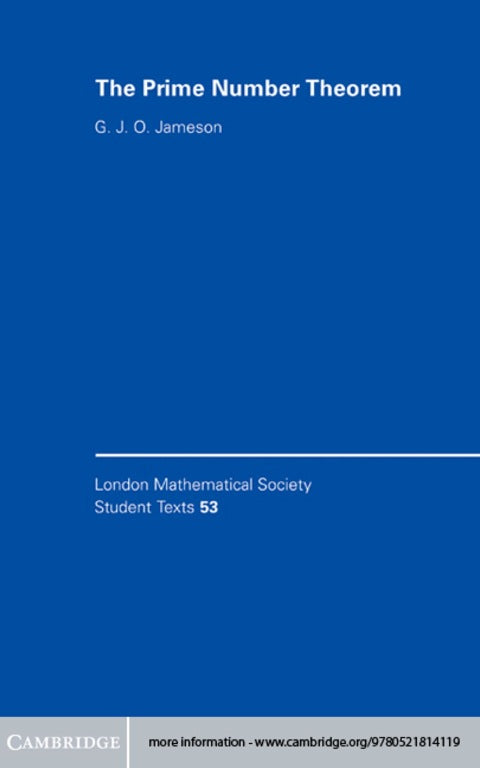# The Prime Number Theorem

G. J. O. Jameson   ·  ISBN 9780511075087Zookal account needed
Get it instantly
\$53.90  Save \$2.68
\$51.22
 Publisher Cambridge University Press Author(s) G. J. O. Jameson Published 04172003 Related course codes
At first glance the prime numbers appear to be distributed in a very irregular way amongst the integers, but it is possible to produce a simple formula that tells us (in an approximate but well defined sense) how many primes we can expect to find that are less than any integer we might choose. The prime number theorem tells us what this formula is and it is indisputably one of the great classical theorems of mathematics. This textbook gives an introduction to the prime number theorem suitable for advanced undergraduates and beginning graduate students. The author's aim is to show the reader how the tools of analysis can be used in number theory to attack a 'real' problem, and it is based on his own experiences of teaching this material.